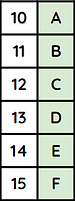## What is binary?

By now you should know that computer systems process data and communicate entirely in binary.

Topic 2.3 explained different binary storage units such as bits (a single 0 or 1), nibbles (4 bits) and bytes (8 bits).

Binary is a base 2 number system. This means that it only has 2 possible values - 0 or 1.

## What is denary?

Denary (also known as decimal) is the number system that you've been using since primary school.

Denary is a base 10 number system. This means that it has 10 possible values - 0, 1, 2, 3, 4, 5, 6, 7, 8, and 9.

## Binary & Denary

Hexadecimal is a base 16 number system. This means that it has 16 possible values - 0, 1, 2, 3, 4, 5, 6, 7, 8, 9, A, B, C, D, E and F.

Hexadecimal is used as a shorthand for binary because it uses fewer characters to write the same value. This makes hexadecimal less prone to errors when reading or writing it, compared to binary. For example, 100111101011 in binary is 9EB in hexadecimal.

Hexadecimal only uses single-character values. Double-digit numbers are converted into letters - use the table on the right to help you understand.## How to convert from hexadecimal to binary:

To convert from denary to hexadecimal or the other way round you must convert to binary first.

Use the videos on this page if you need help converting to or from binary.

The most common number systems question in exams are from denary to hexadecimal or from hexadecimal to denary so make sure that you practice these conversions.

## What is binary shift?

Binary shift is used to multiply and divide binary numbers.

The effect of shifting left is to multiply a binary number.

The effect is doubled by each place that is shifted.

x

The effect of shifting right is to divide a binary number.

÷

Shifting by 1 has an effect of 2.

Shifting by 2 has an effect of 4.

Shifting by 3 has an effect of 8.

For example, shifting left by 2 places has an effect of multiplying by 4.

Another example: Shifting right by 3 places has an effect of diving by 8.

## Questo's Questions

2.4a - Number Systems:

1. Explain why hexadecimal numbers are used as an alternative to binary. Use an example

2. Convert the following values from binary to denary:

• a. 00101010

• b. 11011011

• c. 01011101

• d. 11101110

• e. 01011111     [1 each]

3. Convert the following values from denary to binary:

• a. 35

• b. 79

• c. 101

• d. 203

• e. 250     [1 each]

4. Convert the following values from binary to hexadecimal:

• a. 11110101

• b. 01100111

• c. 10111010

• d. 10010000

• e. 11101001      [1 each]

5. Convert the following values from hexadecimal to binary:

• a. C2

• b. 8A

• c. DE

• d. 54

• e. F7      [1 each]

6. Convert the following values from denary to hexadecimal:

• a. 134

• b. 201

• c. 57

• d. 224

• e. 101     [1 each]

7. Convert the following values from hexadecimal to denary:

• a. 32

• b. A5

• c. 88

• d. C0

• e. BE    [1 each]

Binary Shift:

1a. Draw a diagram to show the effect of multiplying and dividing a binary number. 

1b. Draw a diagram or table to show the effect a shift has for each place from 1 to 4. For example, a shift of 1 place has an effect of 2. 

2. State the effect of the following shifts:

• a. Shift right by 2 places.

• b. Shift left by 1 place.

• c. Shift left 3 places.

• d. Shift right by 4 places.        [1 each]

3. Shift the following binary numbers and state the effect of the shift:

• a. 10101011 : Shift left by 2 places.

• b. 11101100 : Shift right by 3 place.

• c. 00001011 : Shift right by 2 places.

• d. 01101110 : Shift left by 1 place.        [2 each]